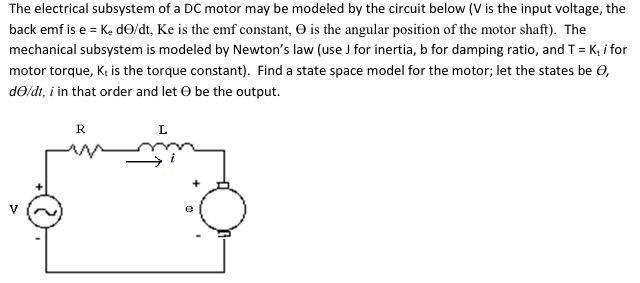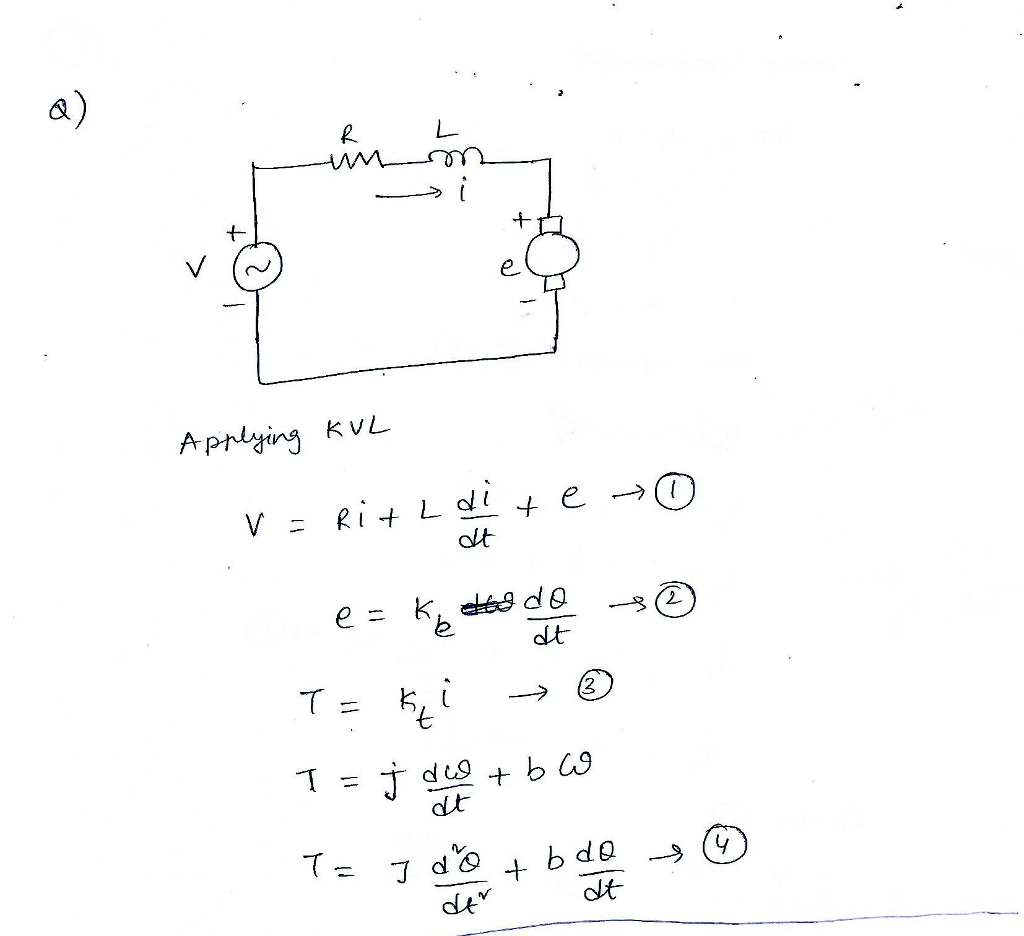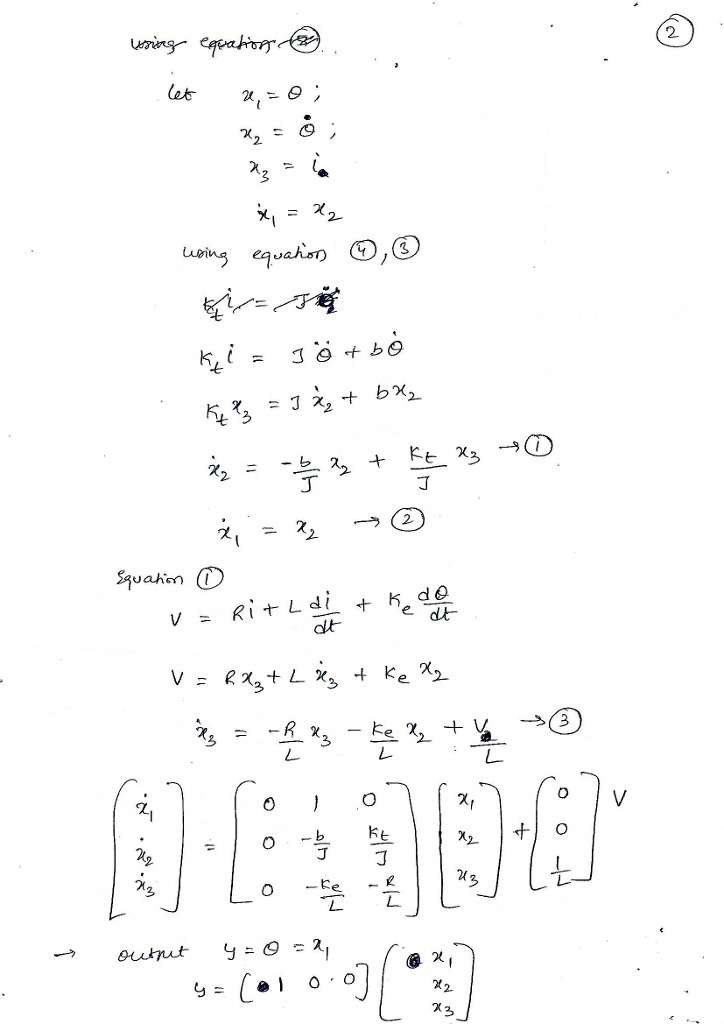# Homework Solution: The electrical subsystem of a DC motor may be modeled by the circuit below (V is the input voltage, the back emf is e = K_e…The electrical subsystem of a DC motor may be modeled by the circuit below (V is the input voltage, the back emf is e = K_e d theta/dt, Ke is the emf constant, theta is the angular position of the motor shaft). The mechanical subsystem is modeled by Newton's law (use J for inertia, b for damping ratio, and T = K_t i for motor torque, K_t is the torque constant). Find a state space model for the motor: let the states be theta, d theta/dt, i in that order and let theta be the output.